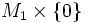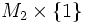# Oriented cobordism of oriented submanifolds

Suppose$M_1$ and$M_2$ are oriented submanifolds of an oriented manifold$N$. An oriented cobordism from$M_1$ to$M_2$ is an oriented submanifold with boundary inside$P \times I$, that gives a cobordism between$M_1 \times \{ 0 \}$ and$M_2 \times \{ 1 \}$ (and such that the orientations match on the boundary).